IV.F Euler Meets The Position, Cost, and Area Problems:
An Introduction to the Fundamental Theorem of Calculus (Best viewed with Netscape 6.0 or higher)
Revision in progress 11-14-2014 to use SAGE and GeoGebra.
Preface: In this section we consider interpretations of Euler's method related to
• the position and velocity of a moving object where the velocity depends only on time,
• the accumulated costs of production where the marginal cost depends only on the level of production, and
• a geometric interpretation (new for this section) that accumulates areas of rectangles. These rectangles all have their bases on the X-axis while their heights depend only the graph of a function y = P(x) lying above that axis.
The important connection between these interpretations is the accumulation of information taken over short intervals of production levels, time, or segments on the X-axis. In using Euler's method we will find the estimates made over small intervals combining to approximate
• the net change of position in the motion interpretation,
• the net change of total cost in the economic interpretation,
• and the area of a planar region in the geometric interpretation.
One major consequence of the commonality of the mathematics underlying these three distinct interpretations is that being able to solve differential equations, i.e., to find indefinite integrals, allows us to determine the area of certain regions in the plane exactly.

Motion and Euler Revisited: As we have seen in IV.E with the motion interpretations of Euler's method, it is commonplace to estimate the change in position of a moving object by multiplying the velocity at a given time and position by the change in time. [Change in Position = velocity times change in time.] For convenience we used the initial time to make these estimates for time intervals of equal duration and then proceeded, step by step, to accumulate the change in position for each of these time intervals.

In the notation of the differential, we estimated the change in position, dSk, during the kth time interval, by multiplying the initial velocity for the kth interval,  S'(xk-1),  by the length of the time interval, dx. So we have dSk = S'(xk-1)dx. (See Figure IV.F.i for the general transformation figure illustration of the differential estimation.) Euler's method is interpreted as the repeated use of this technique to estimate the position changes during short time increments and accumulating the results.

Costs and Euler Revisited: As we have seen in IV.E with the economic interpretations of Euler's method, it is commonplace to estimate the change in cost of a product by multiplying the marginal cost at a given level of productions by the change in level of production. [Change in Cost = marginal cost times units produced.] For convenience we used the initial marginal cost to make these estimates for production intervals of equal quantities and then proceeded, step by step, to accumulate the change in cost for each of these production steps.

In the notation of the differential, we estimated the change in cost, dCk, during the kth production interval, by multiplying the initial marginal cost for the kth interval,  C'(xk-1), by the length of the production interval, dx. So we have dCk = C'(xk-1)dx. (See Figure IV.F.i for the general transformation figure illustration of the differential estimation.) Euler's method is interpreted as the repeated use of this technique to estimate the cost changes during short production increments and accumulating the results.

Note that the discussion of Euler's method in IV.E uses h = (b-a)/N for the change in the controlling variable. We now denote this change with
the differential dx to reinforce the connection with the velocity interpretation.

In summary, if we let dx = (b-a)/N and ak = a + (k-1) dx [so a 0 = a and a N = b] then the estimated change in position for the interval [a,b] based on Euler's method in N steps is the sum,
dS+ dS2 + … + dSn where dSk = S'(ak) dx.

Note that the discussion of Euler's method in IV.E uses h = (b-a)/N for the change in the controlling variable. We now denote this change with the differential dx to reinforce the connection with the marginal cost interpretation.

In summary, if we let dx = (b-a)/N and ak =a + (k-1) dx [ so a0 = a and aN = b] then the estimated change in cost for the interval [a,b] based on Euler's method in N steps is the sum, dC1 + dC2 + … + dCn where dCk = C'(ak) dx.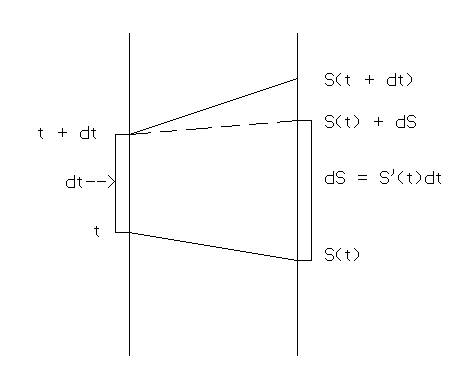Figure IV.F.i
EXAMPLE IV.F.1 ( Motion) : Suppose S is the position of an object moving with the initial position,
S(0) = 5 and
S'(x) = x 2 + 1.
Use Euler's method to estimate S(4) in 4 steps.
Solution: For Euler's method with N = 4 we have h=dx= (4-0)/4= 1. Thus we can compute the estimates using
S(x+dx)≈
S(x) + S'(x)dx
as follows:
(See Figures IV.F.ii.a and b)
 x S(x) ≈ S'(x) = x 2+1 S'(x) dx 0 5 1 1 1 6 2 2 2 8 5 5 3 13 10 10 4 23
S(1) ≈  S(0) + S'(0)1 = 5 + (1)1 = 6
S(2) ≈  S(1) + S'(1)1 = 6 + (2)1 = 8.
S(3) ≈   8 + (5)1 = 13
S(4) ≈  13 + (10)1 = 23.
[Note that since the velocity in this example is always positive, the total position is always increasing. This is consistent with the usual   interpretation of positive velocity.]
1. This work could have been shortened a little by not putting the information together at each step.
Instead of adding the differentials, dS= S'(x)dx, one at a time to the previous estimate, we could accumulate all the differentials to estimate the net change in position and then add that sum to the original position to estimate the final position S(4). The sum of the differentials is 1 + 2 + 5 + 10 = 18. So the estimated change is 18 and the estimate for S(4) is  5 + 18 = 23.

2. To make a more accurate estimate, we could use a larger value for N (more steps) and therefore a smaller value for dx (shorter time intervals).
Thus, with dx = 4/N we estimate S(4) by S(1) plus the sum of the estimated changes of position for the N time intervals of dx.

Using a computer to do the more tedious estimation with N = 100, we find S(4)≈ 30.0144, while with N=1000 the estimate is S(4)≈ 30.1013 . Since the solution for this differential equation is S(t) = t3/3+t+5, we can see that

S(4) = 91/3 = 30 1/3= 30.333
while the exact change in position is S(4) - S(0) = 25 1/3.
EXAMPLE IV.F.1 (Cost):
Suppose C is the cost of producing a commodity with the fixed cost,
C(0) = 5 and
C'(x) = x 2 + 1.
Use Euler's method to estimate C(4) in 4 steps.
Solution: For Euler's method with N = 4 we have h=dx= (4-0)/4 = 1. Thus we can compute the estimates using
C(x+dx)≈
C(x) + C'(x)dx
as follows:
(See Figures IV.F.ii.a and b)

 x C(x) ≈ C'(x) = x 2+1 C'(x) dx 0 5 1 1 1 6 2 2 2 8 5 5 3 13 10 10 4 23
C(1)C(0) + C'(0)1 = 5 + (1)1 = 6.
C(2) C(1) + C'(1)1 = 6 + (2)1 = 8.
C(3) 8 + (5)1 = 13.
C(4) ≈ 13 + (10)1 = 23.
[Note that since the marginal cost in this example is always positive, the total cost is always increasing. This is consistent with the usual interpretation of positive marginal cost.]
: 1. Our work can be shortened a little by not putting the information together at each step.
Instead of adding the differentials, dC= C'(x)dx, one at a time to the previous estimate, we can accumulate all the differentials to estimate the net change in cost and then add that sum to the original cost to estimate the total cost C(4). The sum of the differentials is 1 + 2 + 5 + 10 = 18. So the estimated change is 18 and the estimate for C(4) is 5 + 18 = 23.

2. To make a more accurate estimate, we can use a larger value for N (more steps) and therefore a smaller value for dx (shorter production steps).
Thus, with dx = 4/N we estimate C(4) by C(1) plus the sum of the estimated changes of cost for the N production steps of dx.

Using a computer to do the more tedious estimation with N = 100, we find C(4)≈30.0144, while with N=1000 the estimate is C(4) 30.1013 . Since the solution for this differential equation is C(t) = t3/3+t+5, we can see that

C(4) = 91/3 = 30 1/3= 30.333
while the exact change in cost is C(4) - C(0) = 25 1/3.

 Area:We turn our attention to the concept of area, noting initially that the area of a rectangle is determined as the product of the measurements of the base and the altitude. For a positive function f we can estimate the area of the region enclosed by the graph of the function, the X- axis, the lines X=A and X=B (with A < B) using the area of a rectangle. The rectangle has its base the segment on the X-axis between A and B and its height of length f(A). The area is then estimated by f(A) times (B-A). See Figure IV.F. iii.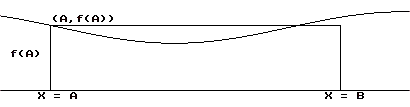If we cut the segment from A to B into smaller segments, each of the same length, dx, we can then consider the areas of the smaller regions that lie above these smaller segments. We still use rectangles to estimate the areas of these smaller regions, but now we must add the estimates to obtain an estimate of the area for the larger region. If we systematically accumulate the areas of the smaller regions, moving along the number line segment by segment, we find that at each step we are adding f(something) times dx to the previous sum. Thus we encounter a process of approximation that is essentially Euler's method, as the next example illuminates further.

EXAMPLE IV.F.2: Estimate the area of the region enclosed by the X-axis, the lines X=1, X=3, and the graph of Y=X 2+1 using Figure IV.F.iv.

SOLUTION: We let A(t) be the area of the region enclosed by the X - axis, the lines X = 1, X = t, and the graph of Y = f(X) = X 2+1 when t >1. Then following the previous ideas, we have

A(t + dx) - A(t) f(t)dx.
or A(t + dx) A(t) + f(t)dx.

See Figure IV.F.v.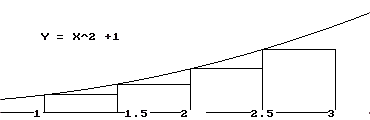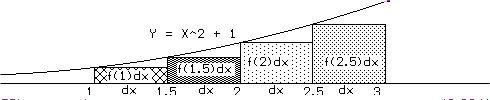Thus we can compute the estimates with dx =.5 as follows: [See Figure IV.F.iv.a]
A(1) = 0 (Given)
A(1.5) A(1) + f(1)dx = 0 + 2(.5) = 1
A(2) A(1.5) + f(1.5)dx1 + 3.25(.5) = 2.625
A(2.5) A(2) + f(2)dx≈ 2.625 + 5(.5) = 5.125
so A(3) A(2.5)+f(2.5)dx≈ 5.125+7.25(.5) = 8.75
 t A(t)≈ f(t) = t 2+1 f(t) dt 1 0 2 1 1.5 1 3.25 1.625 2 2.625 5 2.5 2.5 5.125 7.25 3.625 3 8.75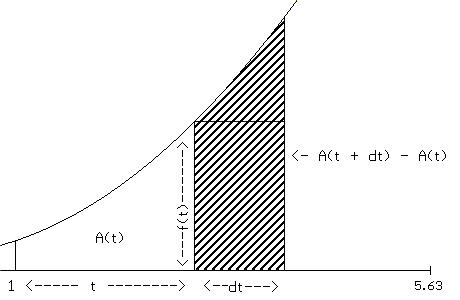What generalizes this method for estimating the area of the region enclosed by the graph of a positive continuous function, the X-axis and two vertical lines? The process is a little simpler than Euler's method because we don't need to keep track of the values of the areas as they accumulate. As we describe this method below, keep in mind the examples we have just completed.

 First, suppose that we want to estimate the area A of the region enclosed by the graph of a positive continuous function Y = P(x), the X - axis, and the lines X = a and X = b. We choose a value for the number of segments of equal length used in the approximation which we denote by N. Then the length of the segments is denoted h or dx = (b-a)/N. We let x 1 = a + dx. We continue by letting x 2 = x 1 + dx [= a + 2dx]. In general (see Figure IV.F.va) we let x k+1 = x k + dx [= a + (k+1)dx], and thus x N = a + Ndx = a + N (b-a)/N = b. For convenience we denote a = x 0.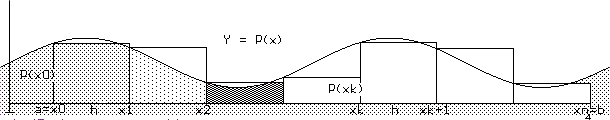Using the vertical lines X = x0, X = x 1, X = x 2, ... , and X = x N = b, the original region is now cut into N pieces . We approximate the area of the entire region by accumulating estimates for the areas of each of these pieces using the areas of rectangles with width dx=h and heights P(x k) where k = 0, 1, 2, ..., N-1. That is, we are using the value of P at the left hand endpoint of each interval to determine the height of the approximating rectangle. Thus we can estimate the area A by

A≈ P(x 0). dx + P(x 1) . dx + P(x 2) . dx +...+ P(x N-1) . dx.
Recognizing the common factor in this last sum gives us the simplest method for estimating the area:
A≈ [P(x0) + P(x1) + P(x2) +...+ P(x N-1)] . dx or
A≈  [P(x0) + P(x1) + P(x2) +...+ P(x N-1)] . h.

EXAMPLE IV.F.3: Estimate the area of the region in the plane enclosed by the X-axis, the lines X =1 and X = 5 and the graph of Y = x 2 + x using Euler's method with N = 4.

Solution: Since N = 4, dx = h = (5 - 1)/4 = 1. In the notation just discussed we have

a = x0 = 1, x1 = 2, x 2 = 3, x 3 = 4, and x 4 = 5 = b.
P(x)= x 2 + x for this problem, so we compute the values of P at the appropriate x's in the table of Figure IV.F.vi .
Thus we have A [P(x 0)+P(x 1)+P(x 2)+P(x 3)] . h. So A [ 2 + 6 + 12 + 20 ] . 1 = 40.
 k x k P(x k) = x k2 + xk 0 1 2 1 2 6 2 3 12 3 4 20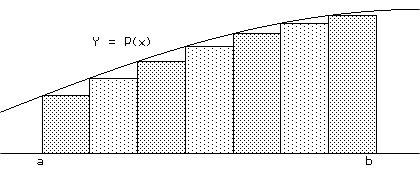Comments: A more accurate estimate of the area might be found by choosing a larger value for N (more intervals) with a resulting shorter line segment for dx. Larger values of N will diminish the size of the corresponding values of dx = h in the calculations. Of course, when N is very large the number of summands and calculations needed for the estimate becomes unwieldy without either a calculator or a computer. But, with appropriate technology available, much greater precision can be obtained in the estimation. As usual we are interested in whether estimate is larger or smaller than the exact area as well as its (relative) precision.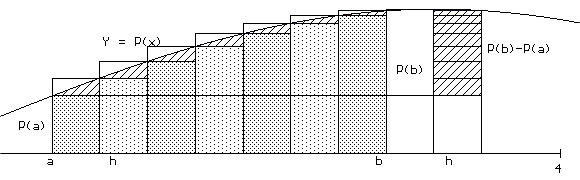From Figure IV.F.vii it should be apparent that if P is an increasing function on the interval [a,b] then the approximation for the area from Euler's method as just outlined will be an underestimate. Similarly, if P is a decreasing function then the approximation will be an overestimate.

Furthermore if P is increasing on the interval [a,b], the difference between the actual area and the estimate obtained by Euler's method is no greater than [P(b)-P(a)].dx.

See Figure IV.F.viii.

A similar statement can be made if P is decreasing on [a,b].

Example IV.F.3 (Continued). On the interval [1,5], P'(t)=2t + 1 is positive, so P(t) = t2 + t is increasing on this interval. We have already found that P(1) = 2 while P(5) = 30. Using N=4 (and therefore dx = h = 1) for our estimate with Euler's method could have resulted in an underestimate by at most (P(5) - P(1)) (1) = 30 - 2 = 28.

You may be thinking at this time, this is not very accurate. To get an estimate that would be guaranteed to be within 1/10 of a square unit of the actual area using Euler's method and our analysis so far, we would have to choose N so that 4/N = h was small enough to make 28h less than 1/10 . That is 28h = 28  4/N < 1/10. Thus we would have to choose a value for N > 4 (28)(10). So any N > 1120 would lead to an estimate for the area that was at most only 1/10 smaller than the area.

This amount of computation is more than you might want to do by hand for the desired accuracy. [In fact a smaller n would do for the same amount of precision. See the excercises.] Here is one place where a computer or a programmable calculator calculator would be helpful. Using a computer with N =1200, the estimate is approximately 53.28, so the actual area should be roughly between 53.28 and 53.38.

 Area meets change in position and velocity The following discussion illustrates how the ability to determine the position (or cost) function from information about the velocity (or marginal cost) function can actually shed light on the area problem. Suppose we are trying to solve the differential equation for the position S(x) of a moving object at time x when the velocity at time x is given by S'(x) = P(x) for some continuous function P, and we are given that the position at x = a is S(a). [We are given an initial position and the velocity.]  Since the velocity is a function of the time only, Euler's method gives a rather easy estimate for the position at x = b where a < b. Letting h = (b-a)/N and a k = a + k h, we obtain the estimate S(b) ≈ S(a) + P(a 0)h + ... + P(a N-1)h. This estimate allows us to estimate the change in the position for the time interval [a,b]. We can find a reasonable estimate for the change in position by accumulating the estimated changes for short time steps of size h, that is S(b) - S(a) ≈  P(a 0)h + ... + P(a N-1)h Figure IV.F.ix visualizes these relations. Area meets change in cost and marginal cost To illustrate how an economics interpretation can add to the understanding of the connection of finding area to solving a differential equation we'll rethink the previous analysis using marginal costs to interpret the derivative.  Suppose we are trying to solve the differential equation for the cost C(x) of producing x units when the marginal cost at production level x is given by C'(x) = P(x) for some continuous function P, and we are given that the cost at x = a is C(a). [We are given a fixed cost and the rate at which the cost is changing.] Since the marginal cost is a function of the level of production alone, Euler's method gives a rather easy estimate for the cost at x = b where a < b. Letting h = (b-a)/N and a k = a + k h, we obtain the estimate C(b) ≈ C(a) + P(a 0)h + ... + P(a N-1)h. This estimate allows us to estimate the change in the production costs for the production interval [a,b]. We can find a reasonable estimate for the change in cost by accumulating the estimated changes for short production steps of size h, that is C(b) - C(a) ≈  P(a 0)h + ... + P(a N-1)h . Figure IV.F.ix visualizes these relations. Area Interpretation: When P(x)>0, the suggested "area" interpretation treats this last sum as an estimate of the area of the region enclosed by the graph of P(x), the lines X=a, X=b, and the X-axis. (See Figure IV.F.x.) Thus if we let A denote the region's area, we have that A≈ [P(a 0)h + ... + P(a N-1)h [≈ S(b) - S(a)]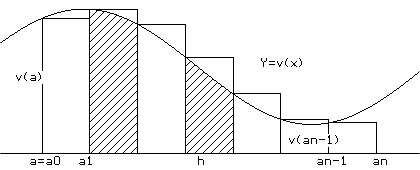By taking n very large (sometimes written n>>0) and thinking of the motion interpretation, we find that the estimating sums must be very close to change in position, S(b) - S(a) where S'(x) = P(x) for x in [a,b]. But from the area interpretation when N>>0 these sums are estimates for the area of the region A.  So the area of the region A must actually equal S(b)-S(a) where S'(x) = P(x). Area Interpretation: When P(x)>0, the suggested "area" interpretation treats this last sum as an estimate of the area of the region enclosed by the graph of P(x), the lines X=a, X=b, and the X-axis. (See Figure IV.F.x.) Thus if we let A denote the region's area, we have that A≈ [P(a 0)h + ... + P(a N-1)h [≈ C(b) - C(a)]By taking n very large (sometimes written n>>0) and thinking of the cost interpretation, we find that the estimating sums must be very close to change in cost, C(b) - C(a) where C'(x) = P(x) for x in [a,b]. But from the area interpretation when N>>0 these sums are estimates for the area of the region A.  So the area of the region A must actually equal C(b)-C(a) where C'(x) = P(x).

The following theorem summarizes the analysis we have made leading to a method for finding an area of a region in the plane using Euler's method with velocity or marginal costs interpretations .

THEOREM IV.3 [Finding the area of a region]: Suppose that
i) P is a positive function continuous on [a,b] and
ii) F is continuous function on the interval with F'(x) = P(x) for all x in [a,b].
Let A be the area of the region enclosed by the graph of P(x), the lines X=a, X=b, and the X-axis

Then A = F(b) - F(a).

Proof: The discussion just completed has the necessary analysis to justify the result.

Comment: Because Theorem IV.3 shows a relation between the derivative of a function and the estimated and exact area of a planar region it is sometimes described as a form of the Fundamental Theorem of Calculus. We will see a more general form of this theorem in Chapter V, called "the evaluation form of the Fundamental Theorem of Calculus."

Example IV.F.4 : Find the area of the region in the plane enclosed by the X- axis, the lines X=1, X=5, and the graph of Y=X 2 +X.

Solution: By applying Theorem IV.3 to the problem, the area of this region is equal to F(5)-F(1) where F'(x) = P(x) = x 2 + x. But it is easy to see that a solution to this differential equation is

F(x) = x 3/3 + x 2/2 + K where K is some constant.
Thus the area of the region is F(5)-F(1) = (53/3 + 52/2 + K) - ( 1 3/3 +  1 2/2  + K)  = 53 1/3.

Another example using GeoGebra with $y'=P(x)$ as a function of $x$ alone:
Estimating  $f (2)$ from  $y' = P( x ) = 2x + 1$  with $f (0) = 0, N = 10 , x_N=2, dx = h = \frac 15 =0.2$.
You can change the values of the end points
on the axis using the sliders or enter them in the boxes in the mapping diagram.
Use the slider to change the value of $N$ up to $100$.
You can change the function definition for the graph and the mapping diagram by entering a new function in the box.
You can change the value of $f(a)$ directly in the spreadsheet cell.

 Martin Flashman, 10 June 2014, Created with GeoGebra

Problems

In problems 1 through 6, use Euler's method with N = 4 (visualized by rectangles) to estimate the area of the region enclosed by the X-axis, the lines X = 1, X = 5 and the graph of P is given. Draw a figure that illustrates the rectangles used in your estimation. Discuss the accuracy of your estimates.

 1. a. P = 3x b. P = 3/x 2. a. P = 5x b. P = 5/x 3. a. P = x 2 b. P = x-2 4. a. P = 50 - x 2 b. P = 50 - x-2 5. a. P = 1 + x 2 b. P = 1/(1 + x 2) 6. a. P = x 2 + x b. P =1/(x 2 + x)
7.-12. For the functions in problems 1 through 6, estimate the area of the region enclosed by the X-axis, the lines X=1, X =3 and the graph of the function using Euler's method with N = 10. Discuss the accuracy of your estimates.

13.-18. For the functions in problems 1 through 6, discuss the use Theorem IV.3 to find the area of the region enclosed by the X-axis, the graph of the function and the lines X = 1 and X = 5. When possible find the area using the theorem. [Comment: At this stage you may find it difficult or impossible to apply this theorem because it is difficult or impossible to find a suitable indefinite integral for P.]

19-24. For the functions in problems 1 through 6, when possible, use Theorem IV.3 to find the area of the region enclosed by the X-axis, the graph of the function and the lines X = 1 and X = 3. [Comment: At this stage you may find it difficult or impossible to apply this theorem because it is difficult or impossible to find a suitable indefinite integral for P.]

25. Sketch a graph of the region enclosed by the X - axis, the lines X = 1, X = 2 and the graph of Y = x 2. Use Euler's method with N = 4 to estimate the area of the region. Discuss the accuracy of your estimate. Find the area of the region using Theorem IV.3.

26. Sketch a graph of the region enclosed by the X-axis, the lines X = 1/2, X=1 and the graph of Y = x 2. Use Euler's method with N = 4 to estimate the area of the region. Discuss the accuracy of your estimate. Find the area of the region using Theorem IV.3.

27. [Uses ln(x)] Sketch a graph of the region enclosed by the X - axis, the lines X = 1, X = 2 and the graph of Y = 1/x. Use Euler's method with N = 4 to estimate the area of the region. Discuss the accuracy of your estimate. Find the area of the region using Theorem IV.3.

28.[Uses ln(x)] Sketch a graph of the region enclosed by the X-axis, the lines X = 1/2, X=1 and the graph of Y = 1/x. Use Euler's method with N = 4 to estimate the area of the region. Discuss the accuracy of your estimate. Find the area of the region using Theorem IV.3.

29. Find the value of t so that the area of the region enclosed by the X-axis, the line X =1, the line X = t (with t>1), and the graph of Y = 3 X 2 is 4.

30. Suppose F'(t) = G'(t) for all t. Prove F(b)-F(a) = G(b)-G(a) for any a and b. [Hint: Use Theorem IV.2.]

31. Give an argument based on Figure IV.F.viii to justify the statement that if P is positive continuous function increasing on the interval [a,b], then the difference between the actual area and the estimate obtained by Euler's method is no greater than [P(b) - P(a)] .h .

32. Draw a figure and give an argument based on your figure to justify the statement that if P is a positive continuous function decreasing on the interval [a,b], then the difference between the actual area of the region enclosed by the X-axis, the lines X = a and X = b and the graph of Y = P(x) and the estimate obtained by Euler's method is no larger than [P(a) - P(b)] .h.

33. Justify the following statements:

a.The difference between the actual area of the region enclosedby the X-axis, the Y-axis, the line X=1 and the graph of Y=1/(X+1) and the estimate for that area obtained by Euler's method when N = 10 is no larger than 1/20.

b.The difference between the actual area of the region enclosed by the X-axis, the Y-axis, the line X=1 and the graph of Y=1/(X 2+1) and the estimate for that area obtained by Euler's method when N = 10 is no larger than 1/20.

34. Find a value of k so that the difference between the actual area of the region enclosed by the X-axis, the Y-axis, the line X=1 and the graph of Y=1/(X +1) and the estimate for that area obtained by Euler's method for N = k is no larger than 1/1000 .

35. Find the value of k so that the difference between the actual area of the region enclosed by the X-axis, the Y-axis, the line X = 1 and the graph of Y = 1/(X+1) and the estimate for that area obtained by Euler's method for N = k is no larger than 10-6 .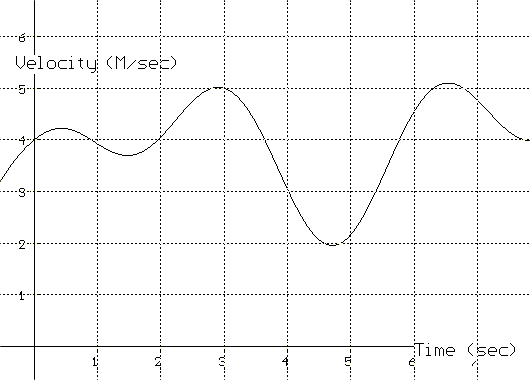36. Suppose the graph in Figure IV.F.xii gives the velocity of a runner in meters per second. If the runner's position after 1 second is 6 meters, estimate the runner's position after 5 seconds using Euler's method with N = 4. Discuss the relation of the runner's position to area.

37. Project: Interpreting Probability with Area.

Recall that the derivative of a probability distribution function for a random variable X is called a probability density function. Consider the dart throwing example of chapter I.C, where the distribution function was given by F(A) = A 2 for A in [0,1] and the density function was given by f(A) = 2A for A in [0,1).

We saw in Chapter I.C that the probability that A<X<B was determined by B 2 - A 2 = F(B) - F(A).

a) Discuss why the probability that A<X<B could be estimated by 2A times (B-A) when B is close to A.

b) Discuss why the probability that A<X<B could be estimated in N steps by partitioning the interval [A,B] into N segments of equal length and then accumulating the probabilities of X being in those segments using the idea of approximation in part a).

c) Draw a figure to illustrate how the probability that A<X<B could be estimated by summing the area of rectangles related to the graph of the probability density function f (A) = 2A.

d) Explain why the probability that A<X<B can be found by finding the area of the region between X = A, X = B, the X-axis, and the graph of the density function Y = f (X) = 2X.

e) Does this make sense in light of Theorem IV.3? Can you generalize the dart example? Explain and justify your generalization.

38. Project: Investment rates and return on investment. Suppose that investment rates of return vary over a 5 year period. Give an interpretation of the area under the graph of the investment rate in terms of the return on an investment of \$1000 over the entire five years of the investment.

39. Project: Concavity and errors in estimating area.
Suppose that P is an increasing positive function on [A,B] and the graph of P is concave up for this interval.
a) Draw a figure for this situation and explain why the difference between P(A) [B-A] and the actual area of the region enclosed by the X-axis, the lines X = A and X = B and the graph of P and is no more than 1/2[ P(B) - P(A)][B-A].

b) Use part a) to explain why the difference between the actual area of the region and the estimate of the area using Euler's method with N segments of equal length will be no more than
[P(B) - P(A)][B-A]/[2N].

c) Use part b) to explain why using N = 561 would be adequate to estimate the area in Example IV.F.3 to within 1/10.

d) Discuss the quality of estimates for decreasing functions that are concave down.

40. Develop a formula for estimating the area of the region enclosed by the X-axis, the lines X = A and X = B, and the graph of a positive valued continuous function based on the use of the value of the function at the right hand endpoint. Draw figures to visualize this situation. Discuss the quality of the estimate in situations when the graph of the function is increasing, decreasing, concave down and concave up.

41. Develop a formula for estimating the area of the region enclosed by the X-axis, the lines X = A and X = B, and the graph of a positive valued continuous function based on the use of the value of the function at the midpoint. Draw figures to visualize this situation. Discuss the quality of the estimate in situations when the graph of the function is increasing, decreasing, concave down and concave up.

0000000000